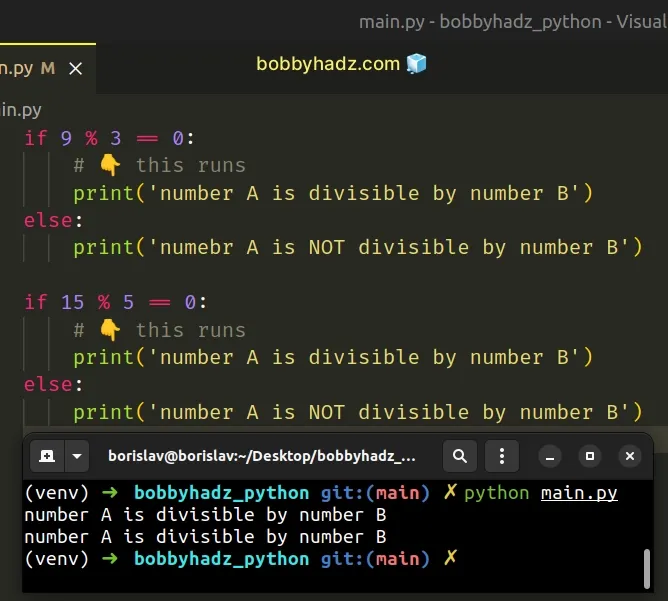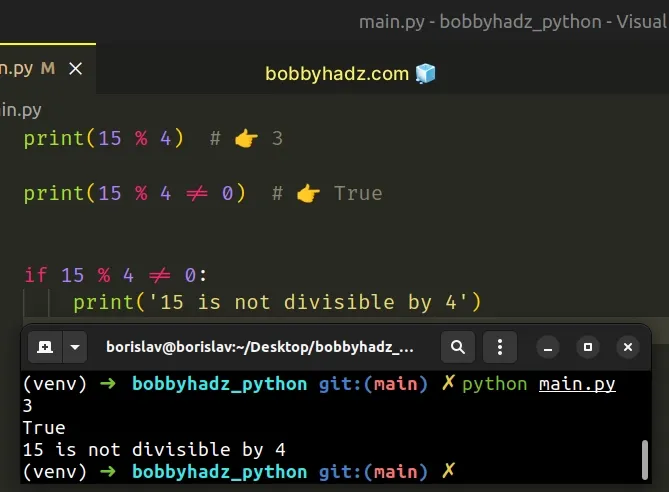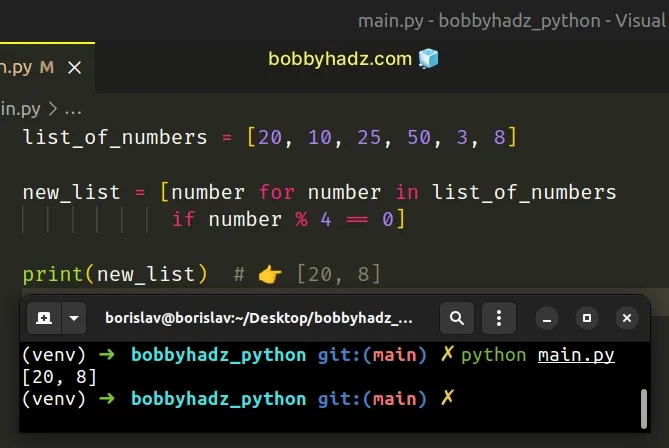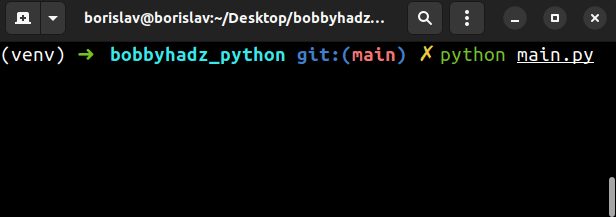# Check if a number is divisible by another number in PythonLast updated: Feb 20, 2023
4 min## #Check if a number is divisible by another number in Python

Use the modulo `%` operator to check if a number is divisible by another number.

The modulo `%` operator returns the remainder from the division of the first number by the second. If the remainder is `0`, the number is divisible by the other number.

main.py
```Copied!```if 9 % 3 == 0:
# 👇️ this runs
print('number A is divisible by number B')
else:
print('numebr A is NOT divisible by number B')

if 15 % 5 == 0:
# 👇️ this runs
print('number A is divisible by number B')
else:
print('number A is NOT divisible by number B')
``````We used the modulo `%` operator to check if a number is divisible by another number.

The modulo (%) operator returns the remainder from the division of the first value by the second.

main.py
```Copied!```print(10 % 2)  # 👉️ 0
print(10 % 4)  # 👉️ 2
``````

If there is no remainder from the division, then the first number is an exact multiple of the second.

main.py
```Copied!```print(16 % 4)  # 👉️ 0

print(16 % 4 == 0)  # 👉️ True
``````

`4` is an exact multiple of `16`, so `16` is divisible by `4` with a remainder of `0`.

## #Checking if a number is not divisible by another number

If you need to check if a number is not divisible by another number, use the modulo `%` operator with the not equals `!=` sign.

main.py
```Copied!```print(15 % 4)  # 👉️ 3

print(15 % 4 != 0)  # 👉️ True

if 15 % 4 != 0:
print('15 is not divisible by 4')
```````15` is not an exact multiple of `4`, so dividing `15` by `4` gives us a remainder of `3`.

## #Find the numbers in a List that are divisible by another number

To find the numbers in a list that are divisible by another number:

1. Use a list comprehension to iterate over the list of numbers.
2. Check if each list item is divisible by the given number using the modulo `%` operator.
3. Return the matching items.
main.py
```Copied!```list_of_numbers = [20, 10, 25, 50, 3, 8]

new_list = [number for number in list_of_numbers
if number % 4 == 0]

print(new_list) # 👉️ [20, 8]
``````List comprehensions are used to perform some operation for every element or select a subset of elements that meet a condition.

On each iteration, we use the modulo operator to check if the current list item is divisible by `4` and return the result.

The new list only contains the matching elements.

## #Find the numbers in a List that are divisible by another number using filter()

Alternatively, you can use the `filter()` function.

main.py
```Copied!```list_of_numbers = [20, 10, 25, 50, 3, 8]

new_list = list(
filter(
lambda x: x % 4 == 0,
list_of_numbers
)
)

print(new_list)  # 👉️ [20, 8]
``````

The filter function takes a function and an iterable as arguments and constructs an iterator from the elements of the iterable for which the function returns a truthy value.

The lambda function we passed to `filter()` gets called with each element from the list.

The function checks if each element is divisible by `4` and returns the result.

The last step is to use the list() class to convert the `filter` object to a list.

## #Check if a Number from user input is divisible by another number

Here is an example that takes numbers from user input and checks if one number is divisible by another.

main.py
```Copied!```num_1 = int(input('Enter a number: '))
print(num_1)  # 👉️ 16

num_2 = int(input("Enter another number: "))
print(num_2)  # 👉️ 4

if num_1 % num_2 == 0:
print(f'{num_1} is divisible by {num_2}')
else:
print(f'{num_1} is NOT divisible by {num_2}')
``````The input function takes an optional `prompt` argument and writes it to standard output without a trailing newline.

Notice that we used the int() class to convert the input strings to integers.

The function then reads the line from input, converts it to a string and returns the result.

Even if the user enters a number, it still gets converted to a string.

## #Check if a number is divisible by two or more numbers

If you need to check if a number is divisible by two or more other numbers, use the `and` operator.

main.py
```Copied!```num = 15

if num % 3 == 0 and num % 5 == 0:
# 👇️ this runs
print('15 is divisible by 3 and 5')
``````

The expression `x and y` returns the value to the left if it's falsy, otherwise the value to the right is returned.

The `if` block is only run if both of the conditions evaluate to `True`.

## #Check if a number is divisible by 1 of multiple numbers

Conversely, if you need to check if a number is divisible by `1` of multiple numbers, use the `or` operator.

main.py
```Copied!```num = 15

if num % 10 == 0 or num % 5 == 0:
# 👇️ this runs
print('15 is divisible by 10 or 5')
``````

The expression `x or y` returns the value to the left if it's truthy, otherwise the value to the right is returned.

If either condition evaluates to `True`, the `if` block is run.